Mobile QR Code1. (Department of Electronics and Electrical Engineering, Dankook University / Yong-in, Korea myoon@dankook.ac.kr)

## 1. Introduction

A multiplier is an essential component of most digital systems, such as microprocessors, digital signal processors, graphic processors, etc. Therefore, various efforts have been made to develop high-speed multipliers [1-6]. The most basic form of multiplication process follows the traditional algorithm taught in primary school and simplifies it to base 2. Multiplication of two $\textit{N}$-digit numbers is composed of three steps. First, $\textit{N}$ partial products are produced by multiplying the multiplicand with each digit of the multiplier. The partial products are then shifted to their proper positions and added by carry save adders (CSA) until only two binary numbers are left in the second step. In the third step, the two numbers are added with a carry propagation adder (CPA) to obtain the final result.

The research on high-speed multipliers focuses on the first and second steps because many high-speed CPAs have been designed and are still researched by adder designers. Booth encoding was developed to reduce the number of partial products. Traditional $\textit{N}$${\times}$$\textit{N}$ multiplication generates $\textit{N}$ partial products, but the radix-$\textit{r}$ ($\textit{r}$=2$^{k}$) Booth multiplier [5-10] can generate one partial product per $\textit{k}$ digits of a multiplier by using encoding logics. Therefore, only $\textit{N}$/$\textit{k}$+1 partial products are generated in the multiplier.

The most critical issue in multiplier design is adding the partial products generated in the first step. Generally, summations with CSAs are performed until two partial sums are left, which should be added by a CPA. The speed of the multiplier depends mostly on the way of reducing the many partial products to two partial sums.

The most widely used reduction scheme is the Wallace-tree , which adds all bits of a digit in parallel with multiple adders. Since tens of bits exist in a digit, several stages of parallel addition are required to obtain the final result. For example, the reduction process for 32 partial products requires 8 stages of adder layers with the Wallace-tree structure. Besides the Wallace-tree architecture, many modified architectures have been researched for fast addition of partial products.

Although many reduction schemes have been developed so far, all multipliers are designed with (3, 2)-adders. Some wide-input adders called adder-compressors  are used for some multiplier designs, but most adder-compressors are also built with (3, 2)-adders. Recently, a (7, 3)-adder based multiplier design was proposed . An ($\textit{m}$, 3)-adder (4 ${\leq}$ $\textit{m}$ ${\leq}$ 7) adds $\textit{m}$ bits in the same digit at a time and produces 3 outputs: S (sum), C (carry), and N (next carry), such that the binary number (N C S) is the result of the addition. A (7, 3)-adder based multiplier uses a (7, 3)-adder as the basic building unit of the multiplier with (6, 3), (5, 3), (4, 3), (3, 2), and (2, 2)- adders as auxiliary units.

The advantage of a (7, 3)-adder based multiplier is that the reduction process with CSA can be built with fewer stages. Although a (7, 3)-adder circuit is slower and more complex than a (3, 2)-adder circuit, the reduced number of stages could compensate for this. In this paper, newly designed fast ($\textit{m}$, 3)-adder (4 ${\leq}$ $\textit{m}$ ${\leq}$ 7) circuits are presented. The new ($\textit{m}$, 3)-adders are smaller, faster and consume less power than previous ($\textit{m}$, 3)-adder circuits.

## 2. (7, 3)-adder based Multiplier Design

All multipliers designed so far use a (3, 2)-adder, which is usually called a full adder for adding partial products. For decades, a number of full adders have been designed, so it may not be possible to design a new faster full adder circuit any more. Therefore, designers of faster multipliers have turned their attention to architectural design of adder layers or efficient parallel processing and pipelining.

To obtain the speed limit of a (7, 3)-adder circuit, it is necessary to compare the number of adder stages in both multipliers. A (3, 2)-adder produces two outputs, S (sum) and C (carry), by adding three bits in the same digit. Since a (3, 2)-adder removes 3 bits by an operation but introduces 2 new bits to the next layer, 1/3 of the number of bits is reduced by a stage. Likewise, an ($\textit{m}$, 3)-adder (4 ${\leq}$ $\textit{m}$ ${\leq}$ 7) adds $\textit{m}$ bits at a time and produces 3 outputs: S, C, and N, so that 4/7 of the number of bits is reduced by a stage of (7, 3)-adder.

Fig. 1 shows the critical path of the reduction process in a 64-bit radix-4 Booth-encoded Wallace-tree multiplier designed with (3, 2)-adders and (7, 3)-adders. 33 partial products can be reduced by three (7, 3)-adder stages and one (3, 2)-adder stage, while 8 stages are required when only (3, 2)-adders are used. Table 1 shows the number of layers required for the reduction step for $\textit{N}$ (=2$^{k}$)-bit Wallace-tree multipliers. To reduce 2$^{k}$(or 2$^{k}$+1) partial products to two partial sums, the (7, 3)-based multiplier needs $\textit{k}$-2 (7, 3)-adder stages and one (3, 2)-adder stage, while the other one requires 2($\textit{k}$-1) stages of (3, 2)-adders. Therefore, the (7, 3)-adders reduce the number of stages by half.

##### Table 1. The Number of Adder Stages Required in the Reduction Process with Wallace-Tree Structure.
 (3, 2)-adder-based (7, 3)-adder-based $\textit{N}$ (2$^{k}$) 32 64 128 32 64 128 $\textit{k}$ 5 6 7 5 6 7 # of partial products 32 64 128 32 64 128 # of (7, 3) stages - - - 3 4 5 # of (3, 2) stages 8 10 12 1 1 1
##### Fig. 1. Critical path for the reduction process of a 64-bit radix-4 Booth multiplier with Wallace-tree architecture: (a) (3, 2)-adder-based multiplier; (b) (7, 3)-adder-based multiplier.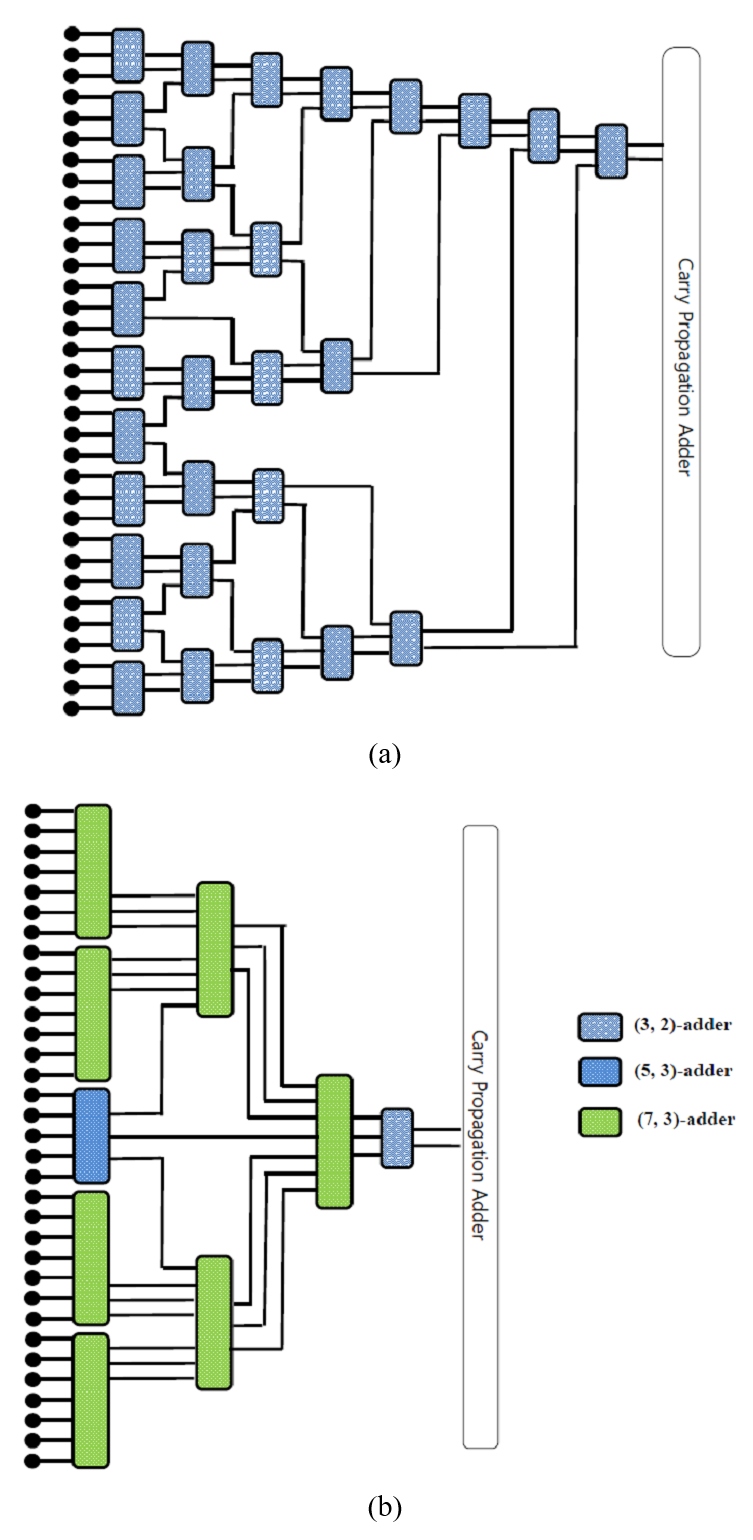For a speed advantage, the delay of a (7, 3)-adder should not exceed 2 times the latency of a (3, 2)-adder. While speed is the most important feature in most circuit designs, power and area are significant features as well.

The number of ($\textit{m}$, 3)-adders required in the reduction step is about 1/3 of the (3, 2)-adders, which could be another criterion for the design of a (7, 3)-adder circuit.

## 3. The Fast (m, 3)-adder Circuits

As the number of inputs increases, the adder circuit becomes slower and more complicated. Therefore, among ($\textit{m}$, 3)-adders, a fast (7, 3)-adder circuit is the bottleneck of the design. It is obvious that the circuits of a (6, 3)-adder, (5, 3)-adder, and (4, 3)-adder will be faster than a (7, 3)-adder circuit if they are designed with the same technique.

For convenience, the newly designed ($\textit{m}$, 3)-adder circuits can be divided into two parts: the body and leaves, as shown in Fig. 2. The circuits for S, C, and N share the same body, and each circuit is generated in a leaf separately by using the outputs of the body. For fast operations, the body is designed with NMOS only. Due to the properties of NMOS, it is strong in pull-down but weak in pull-up. Therefore, there is a large difference between rising and falling delay: the rising delay is very large, whereas the falling delay is small.

The main idea for the circuit design is that the outputs S, C, and N should respond only to negative transitions. For example, the sum of 7 inputs, S, is 1 when X1 or X3 is 0, but it is 0 otherwise. Assume that the inputs change from (0000000) to (0000111) and then to (0000101). (X0, X1, X2, X3) will be changed from (0111) to (1110) and to (1101). Initially, S is 0 and is then changed to 1 since X3 becomes 0. S is changed quickly since the falling delay of X3 is small. Next, X3 becomes 1, so S should be changed to 0. If S is controlled by X1 and X3 only, S should be changed after X3 becomes 1, which is very slow compared to negative transition. To avoid the influence of positive transitions, let S be controlled by not only X1 and X3, but also X0 and X2. In the second transition, S is controlled by the negative transition of X2 instead of the positive transition of X3. The circuit in Fig. 2(c) makes the output respond to changing signals such that S is changed by X2 even if both X2 and X3 are 0 for a while.

C and N circuits work in the same manner. The same design technique is applied for other ($\textit{m}$, 3)-adder circuits. The circuits of (4, 3), (5, 3), (6, 3), and (7, 3)-adders are presented in Figs. 2-5. The leaf circuits of S and C are the same for all ($\textit{m}$, 3)-adder circuits.

##### Fig. 2. The circuit of a (4, 3)-adder: (a) body part; (b) leaf for N circuit; (c) leaf for S circuit; (d) leaf for C circuit.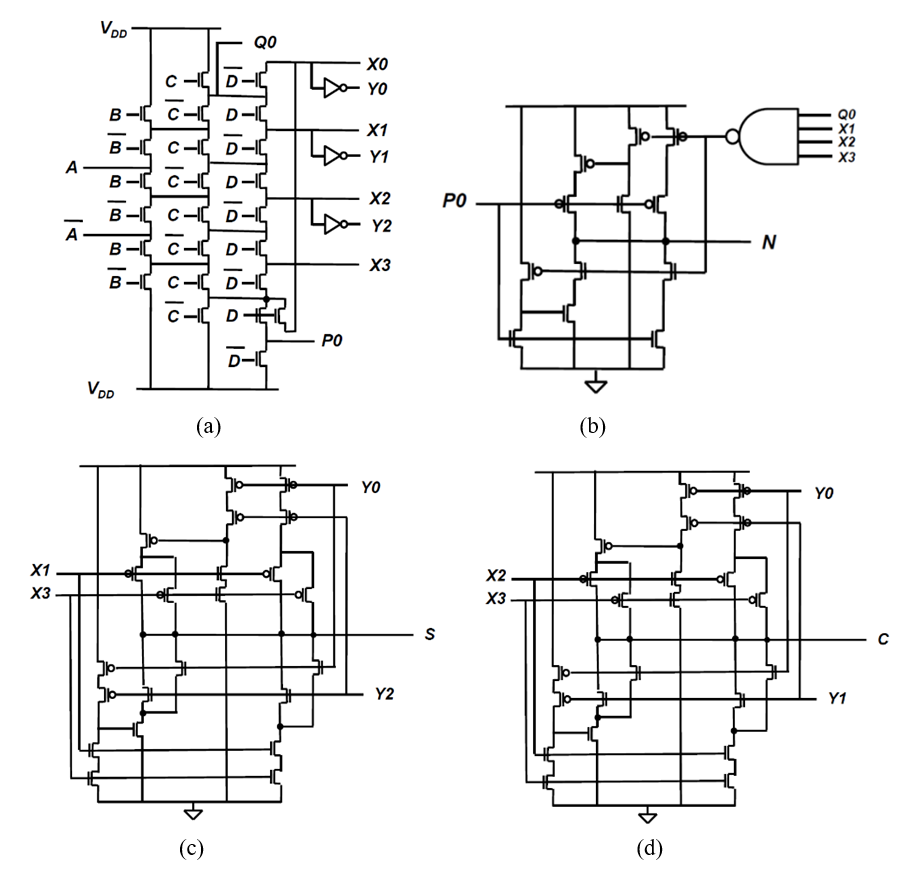##### Fig. 3. The circuit of a (5, 3)-adder: (a) body part; (b) leaf for N circuit.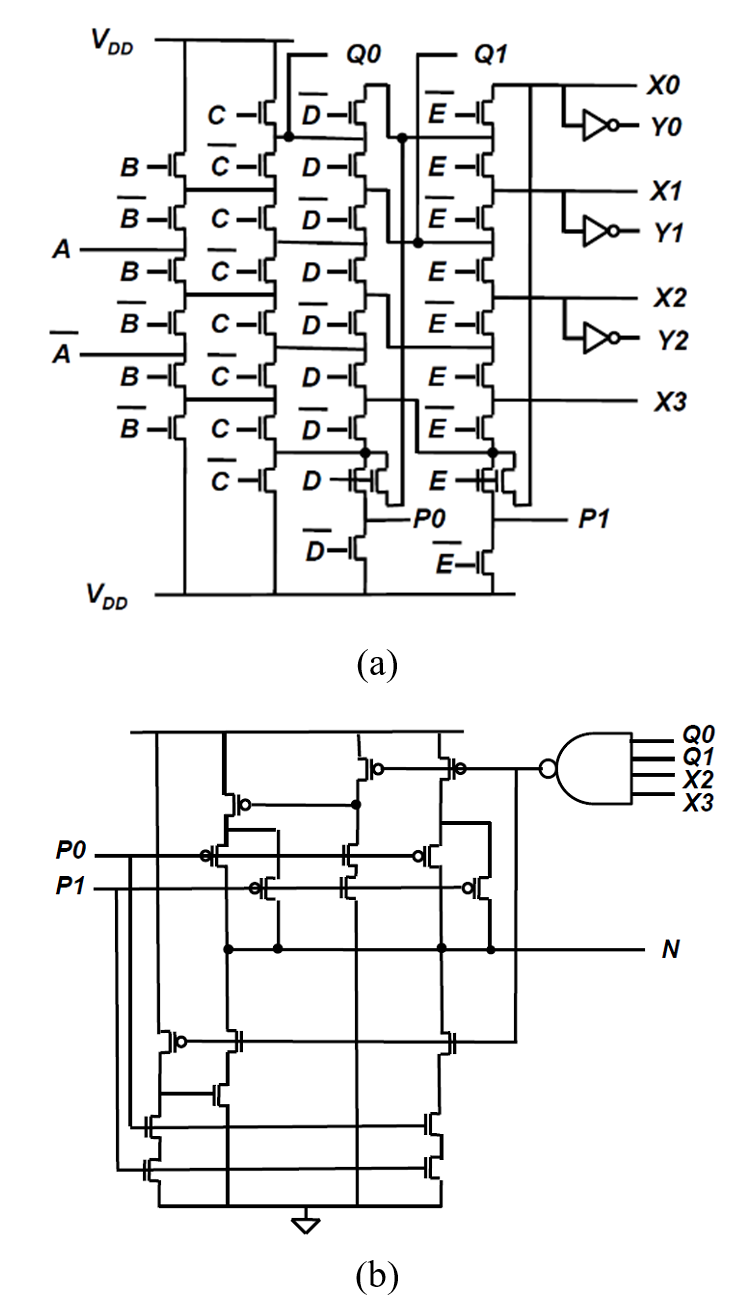##### Fig. 4. The circuit of a (6, 3)-adder: (a) body part; (b) leaf for N circuit.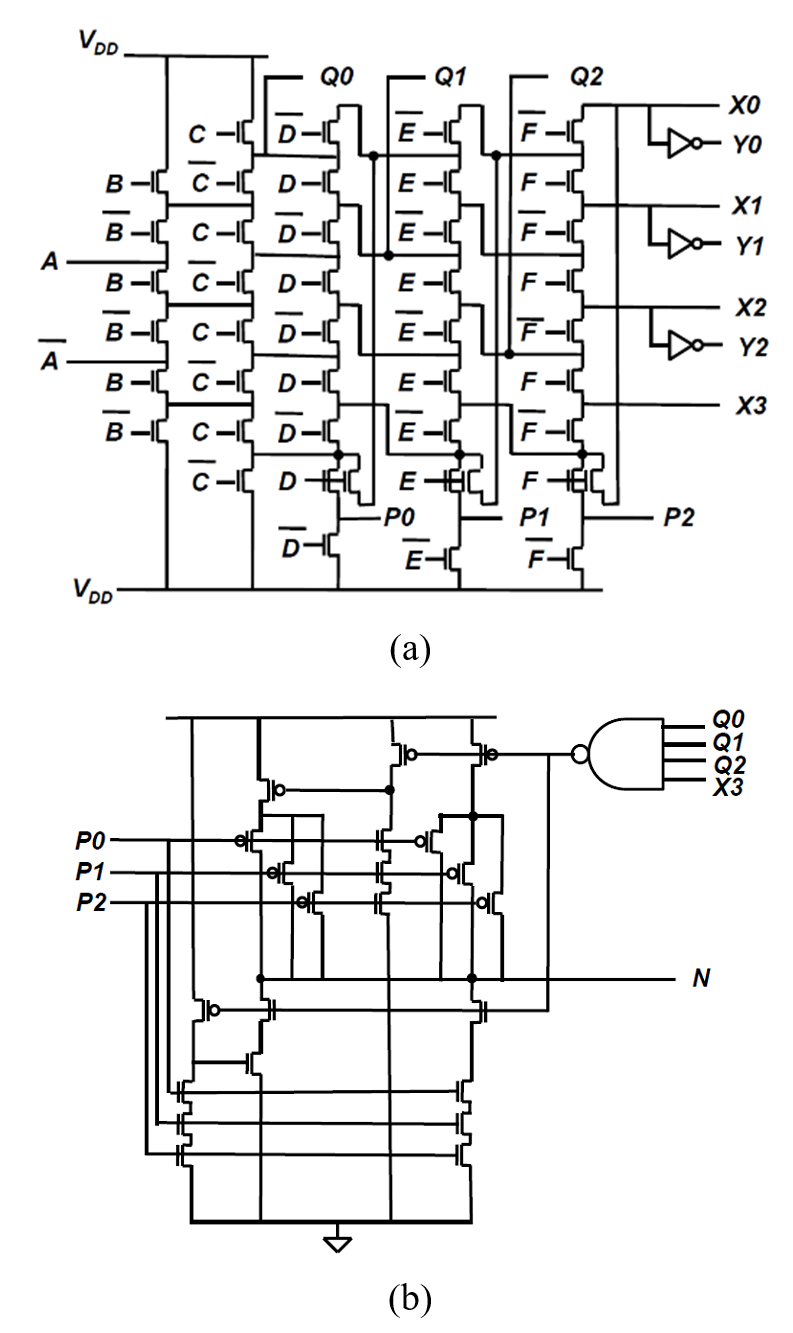##### Fig. 5. The circuit of a (7, 3)-adder: (a) body part; (b) leaf for N circuit.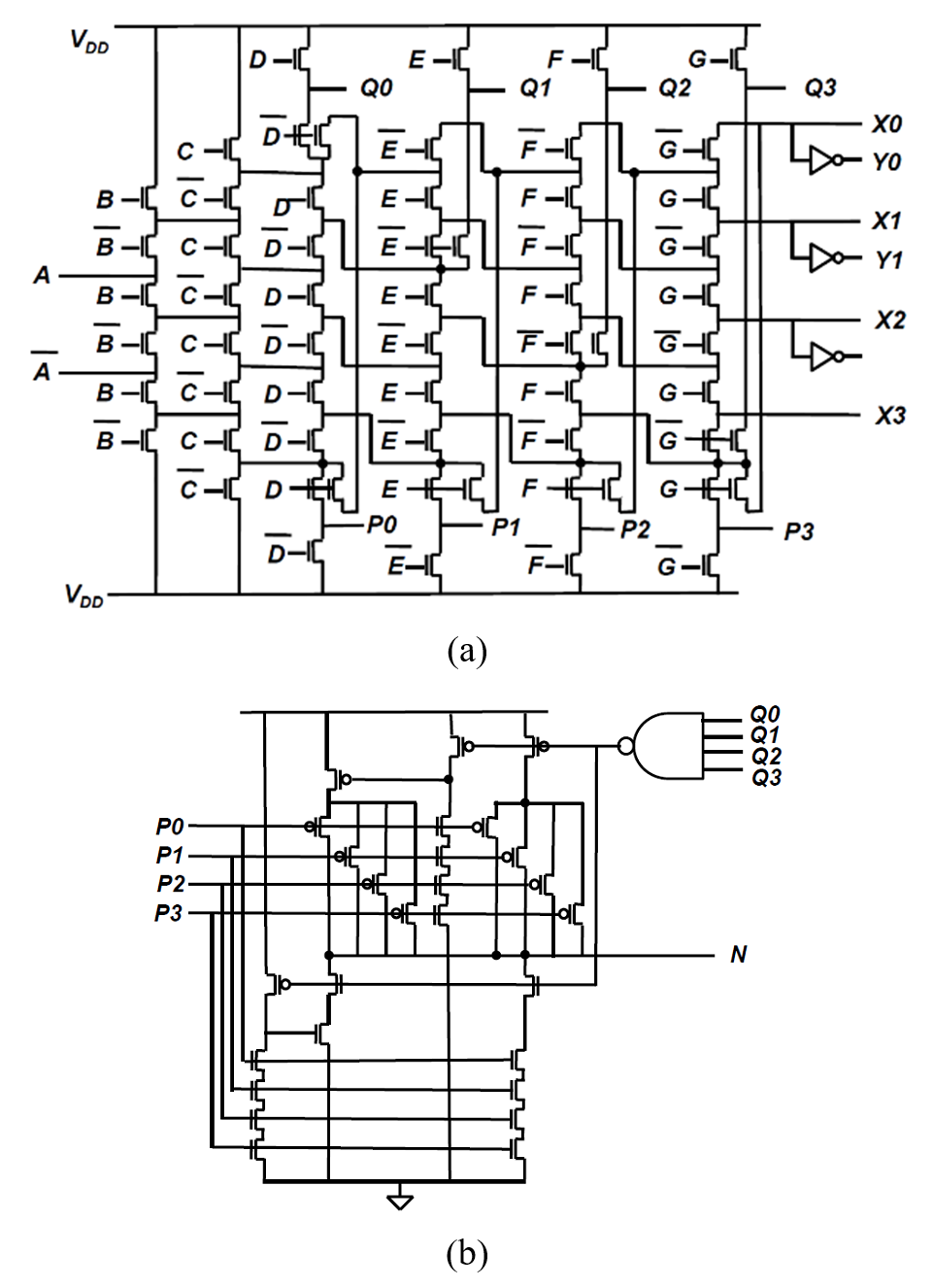## 4. Experiments

The characteristics of the newly designed ($\textit{m}$, 3)-adders were estimated by simulations and compared with those of existing ($\textit{m}$, 3)-adder circuits . The simulation was performed by HSPICE with 1.2V-0.13${\mathrm{\mu}}$m model parameter.

Table 2 summarizes the simulation results comparing the speed of the newly designed circuits with the existing circuits  and the reference (3, 2)-adder circuit. The well-known full adder circuit as in Fig. 6  is chosen as the reference (3, 2)-adder circuit. The delay of an adder is measured by cascading 10 identical adders (fanout=1). The delays and power of the circuits are measured in various combinations of input changes. The speed of the (7, 3)-adder varies widely with the change of inputs. The worst case is when input A changes, while the best case is when only input G changes. The delay and the power in Table 2 are taken as the worst-case values in the simulations.

##### Fig. 6. The reference (3, 2)-adder circuit.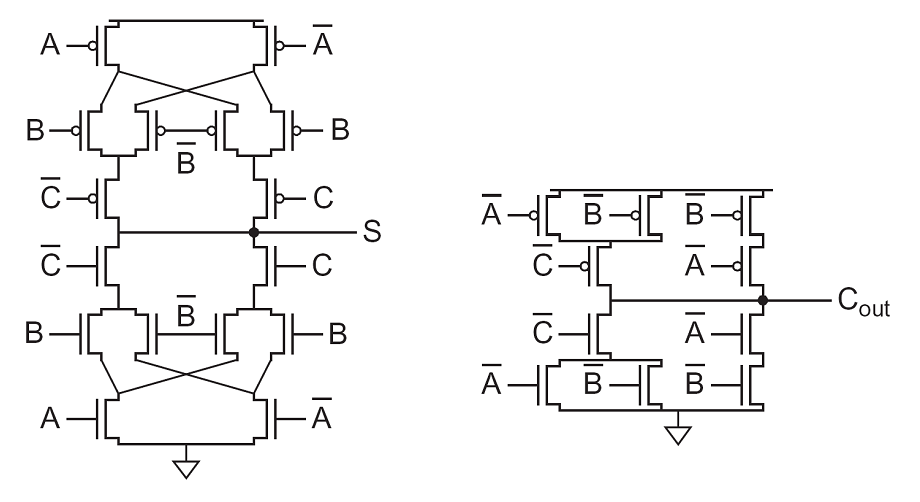##### Table 2. Simulation Results.
 Adder (3, 2) (4, 3) (5, 3) (6, 3) (7, 3) Ref. 280 In  315 381 422 528 New 312 319 352 406 Ref. 5.66 New 8.20 9.31 10.81 13.43
* Power is measured at 100-MHz frequency

To estimate the speed enhancement of the (7, 3)-adder based multiplier, 64-bit radix-4 Booth multipliers were designed based on the (3, 2)-adder and the (7, 3)-adder. Since the (7, 3)-adder is used only in the reduction step, the two multipliers differ only in the reduction circuit. The same radix-4 Booth encoder circuit and 128-bit carry selection adder are used in both multipliers. The delays of the multipliers are simulated with HSPICE, and the result is shown in Table 3.

By using the new (7, 3)-adder circuit, the (7, 3)-adder based multiplier completes the reduction step 35% faster than the (3, 2)-adder based Wallace-tree reduction circuits. Enhancement of the total delay depends largely on the 128-bit adder used in the multiplier because many adders have been developed, and their delays differ widely. In the simulation, a progressively partitioned carry selection adder  was used in the multiplier. The latency of the adder is 3.2 ns for addition of two 128-bit binary numbers. With this 128-bit adder, the (7, 3)-adder-based multiplier is 13% faster than the (3, 2)-adder based multiplier. Recently, a 128-bit modified carry look-ahead adder  was proposed, and its delay was 0.42 ns with 1.8V-0.18${\mu}$m technology. If this adder is used, the speed is increased by 25%. For a speed-oriented design, in general, a high-speed adder is employed in the CPA step, so the latency of the reduction step would be the dominant component of the total delay. Therefore, the efficiency of the (7, 3)-adder increases when a high-speed 128-bit adder is used.

##### Table 3. The Delay Components of a 64-bit Radix-4 Booth Multiplier.
 Delay (ns) (3,2)-based (7,3)-based Partial product generation 0.54 0.54 Reduction (CSA) 2.24 1.45 Carry propagation addition 3.20 3.20 Total 5.98 5.19
##### Table 4. Comparison of the Number of CSA Adders to Build a 64-bit Radix-4 Booth Multiplier.
 Adder Wallace-Tree (7, 3) based # of adders (2, 2) 67 26 (3, 2) 2021 218 (4, 3) - 95 (5, 3) - 100 (6, 3) - 102 (7, 3) - 378 # of stages (7, 3) 3 (3, 2) 8 1

The simulation results verify that a faster and lower-power multiplier can be implemented by employing the (7, 3)-adder. The design of wider-input adders could serve as an alternative approach for high-speed multipliers.

## 5. Conclusion

A set of ($\textit{m}$, 3)-adder circuits was presented in this paper for use in the design of a high-speed multiplier. Simulations proved that the (7, 3)-adder based multiplier with the proposed circuits is faster and uses less power than the (3, 2)-adder based multiplier.

The circuit of a (3,2)-adder is relatively simple, and various circuits have been developed for decades, so it is almost impossible to design a new faster circuit. However, the circuit of a (7, 3)-adder is complex and rarely developed. The proposed adder circuits verified that high-performance multipliers can be achieved through the design of a high-performance (7, 3)-adder circuit.

### REFERENCES

1
Park M. C., Lee B. W., Kim G. M., Kim D. H., 1993, Compact and Fast Multiplier Using Dual Array Tree Structure, IEEE Int. Symp. on Circuit and Systems, Chigago, Vol. 3, pp. 1817-18202
Wallace C.S., 1964, A Suggestion for a Fast Multiplier, IEEE Trans. Electron. Compt., Vol. 13, No. 1, pp. 14-173
Dadda L., 1965, Some Schemes for Parallel Multipliers, Alta Frequenza4
Mehta P., Gawali D., 2009, Conventional versus Vedicmathematical method for Hardware implementation of a multiplier, IEEE Int. Conf. on Advances in Computing, Control, Telecommun.Technologies, pp. 640-6425
Booth A. D., 1951, A Signed Binary Multiplication Technique, Jour. ofMech. Appl. Math., Vol. 4, pp. 236-2406
Yeh W. C., Jen C. W., 2000, High-Speed Booth Encoded Parallel Multiplier Design, IEEE Trans. on Compt., Vol. 49, No. 7, pp. 692-7017
Schwarz E. M., III R. M. A., Sigal L. J., 1997, A radix-8 CMOS S/390 multiplier, in Proc. 13th IEEE Symp. Comput. Arithmetic (ARITH), pp. 2-98
Colon-Bonet G., Winterrowd P., 2008, Multiplier evolution: A familyof multiplier VLSI implementations, Comput. J., Vol. 51, No. 5, pp. 585-5949
Riedlinger et al. R., 2012, A 32 nm, 3.1 billion transistor, 12 wide issue itanium processor for mission-critical servers, IEEE J. Solid-State Circuits, Vol. 47, No. 1, pp. 177-19310
Rao M., Dubey S., 2012, A high speed and area efficient Booth recoded Wallace tree multiplier for fast arithmetic circuits, Microelectronics and Electronics (Prime Asia), 2012 Asia Pacific Conference onPostgraduate Research, pp. 220-22311
Yoon M., 2019, Design of A Fast Multiplier with (m, 3)-Adders, IJERT, Vol. 12, No. 10, pp. 1757-1763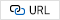12
Neil H.E. Weste , David M. Harris , Kamran , 2010, Principles of CMOS VLSI Design: A systems perspective, Pearson, Fourth Edition13
Pilato L., Saponara S., Fanucci L., 2016, Performance of digital adder architectures in 180nm CMOS standard-cell technology, 2016 International Conference on Applied Electronics (AE), pp. 211-21414
Ghafari S., Mousazadeh M., Khoei A., Dadashi A., 2019, A New High-speed and Low area Efficient Pipelined 128-bit Adder Based on Modified Carry Look-ahead Merging with Han-Carlson Tree Method, 2019 MIXDES - 26th International Conference "Mixed Design of Integrated Circuits and Systems", pp. 157-162## Author

Myungchul Yoon received the BS and MS degrees in Electronics Engineering from Seoul National University, Korea, in 1986 and in 1988 respectively, and the Ph.D. degree in Electrical and Computer Engineering from the University of Texas at Austin in 1998. From 1988 to 2002, he was with Hynix Inc. Icheon, Korea as a technical research staff at Semiconductor R&D Lab., and Mobile Communication R&D Lab. From 2005 to 2006, he was with DGIST, Korea as a technical staff at the Information Technology R&D Division. Since 2006, he has been with the Department of Electronics and Electrical Engineering, Dankook University, YongIn, Korea, where he is a professor. His research interests are in low-power VLSI design, embedded systems, and mobile communication..